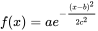# Wand gaussian_blur() function in Python

Another type of blur is Gaussian Blur. Difference of Gaussian blur with normal is that Gaussian Blur is achieved by using Gaussian Function. Simply any equation of the form :is called Gaussian Function.

Syntax :

## Python3

 `wand.image.gaussian_blur(radius``=``"radius_value", ` `                           ``sigma``=``"sigma_value", ` `            ``channel ``=` `"optional_channel_value")`   `# radius should always be greater than sigma(standard deviation)`

Parameters :

Image Used :Example #1:

## Python3

 `# import display() to show final image` `from` `wand.display ``import` `display`   `# import Image from wand.image module` `from` `wand.image ``import` `Image`   `# read file using Image function` `with Image(filename ``=``"koala.jpeg") as img:`   `    ``# perform gaussian blur effect using gaussian_blur() function` `    ``img.gaussian_blur(radius ``=` `5``, sigma ``=` `4``)`   `    ``# save final image` `    ``img.save(filename ``=``"gb_koala.jpeg")`   `    ``# display final image` `    ``display(img)`

Output :Example #2: Reduced radius and sigma

## Python3

 `# import display() to show final image` `from` `wand.display ``import` `display`   `# import Image from wand.image module` `from` `wand.image ``import` `Image`   `# read file using Image function` `with Image(filename ``=``"koala.jpeg") as img:`   `    ``# perform gaussian blur effect using gaussian_blur() function` `    ``img.gaussian_blur(radius ``=` `2``, sigma ``=` `3``)`   `    ``# save final image` `    ``img.save(filename ``=``"gb_koala.jpeg")`   `    ``# display final image` `    ``display(img)`

Output :Whether you're preparing for your first job interview or aiming to upskill in this ever-evolving tech landscape, GeeksforGeeks Courses are your key to success. We provide top-quality content at affordable prices, all geared towards accelerating your growth in a time-bound manner. Join the millions we've already empowered, and we're here to do the same for you. Don't miss out - check it out now!

Previous
Next